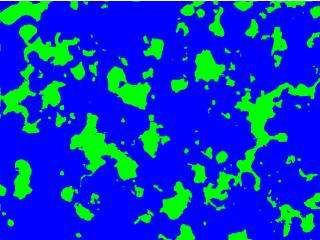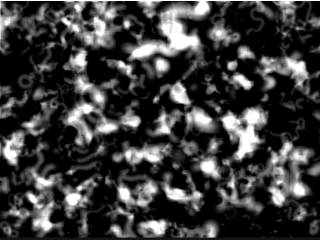-=+=- -=+=- -=+=- -=+=- -=+=- -=+=- -=+=- -=+=- -=+=- -=+=- -=+=- -=+=- -=+=- -=+=- -=+=- -=+=- -=+=- -=+=- -=+=- -=+=- -=+=- -=+=- -=+=- -=+=- -=+=- -=+=- -=+=- -=+=- -=+=- -=+=- (c) WidthPadding Industries 1987 0|494|0 -=+=- -=+=- -=+=- -=+=- -=+=- -=+=- -=+=- -=+=- -=+=- -=+=- -=+=- -=+=- -=+=- -=+=- -=+=- -=+=- -=+=- -=+=- -=+=- -=+=- -=+=- -=+=- -=+=- -=+=- -=+=- -=+=- -=+=- -=+=- -=+=- -=+=-
SoCoder -> Snippet Home -> Generators

PakzCreated : 21 January 2019
Edited : 21 January 2019
Language : Monkey2

### Maps or oilish paintings

Smearing a additive brush

Screenshots## Latest Update

Here I have coded a routine that uses a simulated brush. I stroke it into random directions and modify the angle somewhat during the stroke.

Additive is where you add a number ontop of another one. This can be used for blending etc.

The results of the code is either a simplified world map or a oilish painting.